# 手把手教你移植一个麦语言策略（进阶）

``````(*backtest
start: 2019-05-01 00:00:00
end: 2019-11-12 00:00:00
period: 1d
exchanges: [{"eid":"Futures_OKCoin","currency":"BTC_USD"}]
args: [["SlideTick",10,126961],["ContractType","quarter",126961]]
*)

N1:=10;
N2:=21;
AP:=(HIGH+LOW+CLOSE)/3;
ESA:=EMA(AP,N1);
D:=EMA(ABS(AP-ESA),N1);
CI:=(AP-ESA)/(0.015*D);
TCI:=EMA(CI,N2);
WT1:TCI;
WT2:SMA(WT1,4,1);
AA:=CROSS(WT1,WT2);
BB:=CROSSDOWN(WT1,WT2);
REF(AA,1),BPK;
REF(BB,1),SPK;
``````

• EMA
该指标函数，在FMZ平台用JavaScript语言编写策略时直接有现成的指标库函数。即：`TA.MA`

• SMA
需要我们动手的是`SMA`这个指标，我们发现在FMZ的TA库中没有支持SMA这个指标函数，在talib库中SMA指标和麦语言中的也有差别：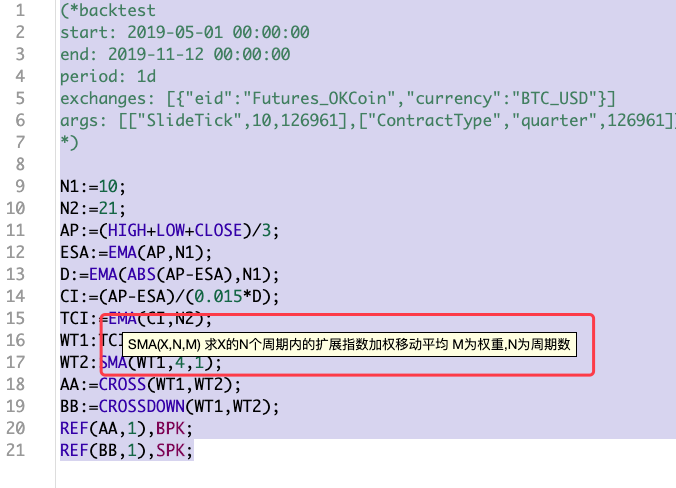可以看到，参数部分除了周期参数，还有一个权重参数。

FMZ API文档中talib库中SMA指标函数的描述为：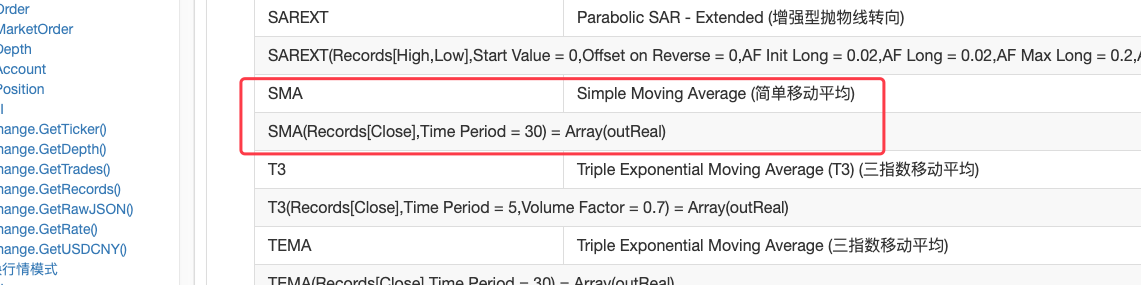可见`talib.SMA`是一个简单移动平均指标。
这样就只能动手自己实现一个`SMA`了，作为使用JavsScript语言编写策略的开发者，这也是必备技能之一，毕竟如果没有现成的轮子，车还是要开的，造一个就是了。

说实话，对于指标之类的研究不多，一般都是不懂的就搜索，查资料。对于SMA查到这些：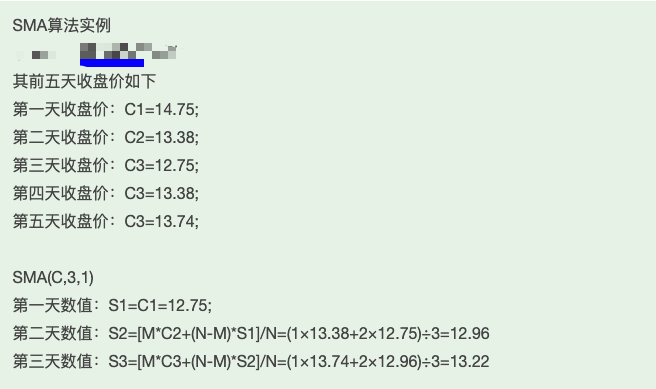感觉这个说的算法过程挺靠谱，实现一下：

``````function SMA (arr, n, m) {
var sma = []
var currSMA = null
for (var i = 0; i < arr.length; i++) {
if (arr[i] && !isNaN(arr[i])) {
if (!currSMA) {
currSMA = arr[i]
sma.push(currSMA)
continue
}

// [M*C2+(N-M)*S1]/N
currSMA = (m * arr[i] + (n - m) * currSMA) / n
sma.push(currSMA)
} else {
sma.push(NaN)
}
}

return sma
}
``````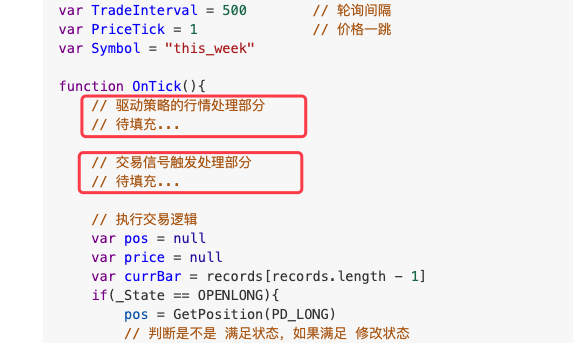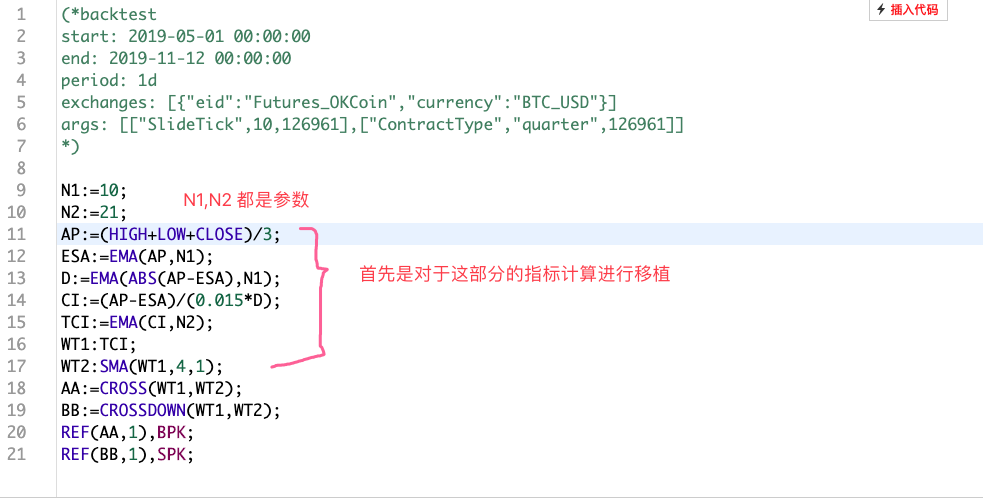• 1、`AP:=(HIGH+LOW+CLOSE)/3;`

这句可以理解为，要把K线数据中的每根BAR的最高价、最低价、收盘价相加再除以3，计算平均值，然后存为一个数组，和每个BAR一一对应。
可以这样处理：

``````function CalcAP (r) {   // AP:=(HIGH+LOW+CLOSE)/3;
var arrAP = []      // 声明一个空数组

for (var i = 0; i < r.length; i++) {      // r为传入的K线数据，是一个数组，用for遍历这个数组
v = (r[i].High + r[i].Low + r[i].Close) / 3      // 计算 平均值
arrAP.push(v)                                    // 添加在 arrAP数组的尾部，arrAP是空的时候尾部就是第一个。
}

return arrAP     // 返回 这个平均值数组，即麦语言中计算的 AP
}
``````

在主循环OnTick函数中调用这个函数就可以了，例如：

``````// 计算指标
// AP
var ap = CalcAP(records)
``````
• 2、AP计算完成以后，接着计算`ESA:=EMA(AP,N1);`:

这里要使用上一步算出的AP这个数据，计算ESA，其实这个ESA就是AP的「指数移动平均」，即EMA指标，所以我们就用AP作为数据，N1作为EMA指标的参数，计算EMA指标就可以了。

``````function CalcESA (ap, n1) {   // ESA:=EMA(AP,N1);
if (ap.length <= n1) {    // 如果AP的长度小于指标参数，是无法计算出有效数据的，这个时候让函数返回false。
return false
}

return TA.EMA(ap, n1)
}
``````
• 3、`D:=EMA(ABS(AP-ESA),N1);`

使用计算出的`AP``ESA`计算数据`D`
此处代码注释可以看下，有些指标计算的技巧。

``````function CalcD (ap, esa, n1) {    // D:=EMA(ABS(AP-ESA),N1);
var arrABS_APminusESA = []
if (ap.length != esa.length) {
throw "ap.length != esa.length"
}

for (var i = 0; i < ap.length; i++) {
// 计算指标数值时，必须判断一下数据的有效性，因为前几次EMA计算可能数组中的开始部分的数据是NaN,或者null
// 所以必须判断，参与计算的数据都是有效数值才能进行，如果有任何无效数值，就用NaN向arrABS_APminusESA填充
// 这样计算得到的数据，每个位置和之前的数据都是一一对应的，不会错位。
if (ap[i] && esa[i] && !isNaN(ap[i]) && !isNaN(esa[i])) {
v = Math.abs(ap[i] - esa[i])     // 根据ABS(AP-ESA) , 具体计算数值,然后放入arrABS_APminusESA数组
arrABS_APminusESA.push(v)
} else {
arrABS_APminusESA.push(NaN)
}
}

if (arrABS_APminusESA.length <= n1) {
return false
}

return TA.EMA(arrABS_APminusESA, n1)    // 计算数组arrABS_APminusESA的EMA指标，得到数据D（数组结构）
}
``````
• 4、`CI:=(AP-ESA)/(0.015*D);`
这个计算方式和步骤1类似，直接放出代码。

``````function CalcCI (ap, esa, d) {    // CI:=(AP-ESA)/(0.015*D);
var arrCI = []
if (ap.length != esa.length || ap.length != d.length) {
throw "ap.length != esa.length || ap.length != d.length"
}
for (var i = 0; i < ap.length; i++) {
if (ap[i] && esa[i] && d[i] && !isNaN(ap[i]) && !isNaN(esa[i]) && !isNaN(d[i])) {
v = (ap[i] - esa[i]) / (0.015 * d[i])
arrCI.push(v)
} else {
arrCI.push(NaN)
}
}

if (arrCI.length == 0) {
return false
}

return arrCI
}
``````
• TCI:=EMA(CI,N2);
只是计算CI数组的EMA指标。

``````function CalcTCI (ci, n2) {   // TCI:=EMA(CI,N2);
if (ci.length <= n2) {
return false
}

return TA.EMA(ci, n2)
}
``````
• WT2:SMA(WT1,4,1);

最后这步骤，用到了我们之前造好的轮子`SMA`函数。

``````function CalcWT2 (wt1) {    // WT2:SMA(WT1,4,1);
if (wt1.length <= 4) {
return false
}

return SMA(wt1, 4, 1)   // 使用我们自己实现的SMA函数计算出wt1的SMA指标。
}
``````

``````AA:=CROSS(WT1,WT2);
BB:=CROSSDOWN(WT1,WT2);
REF(AA,1),BPK;
REF(BB,1),SPK;
``````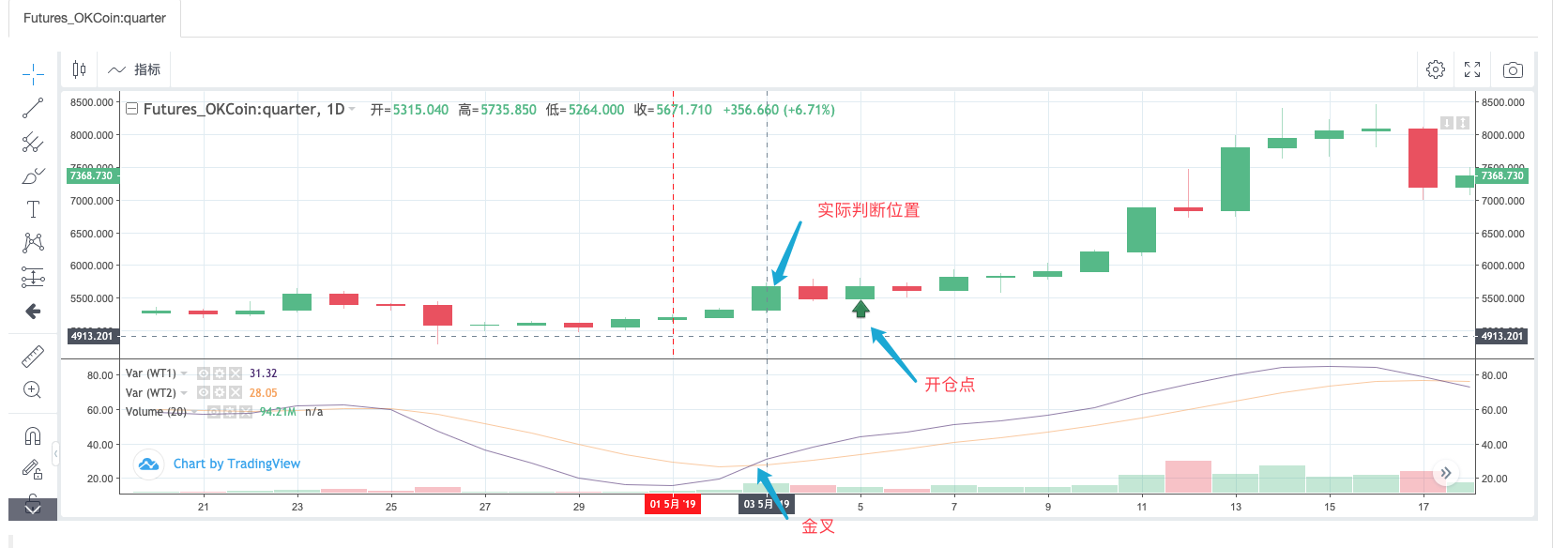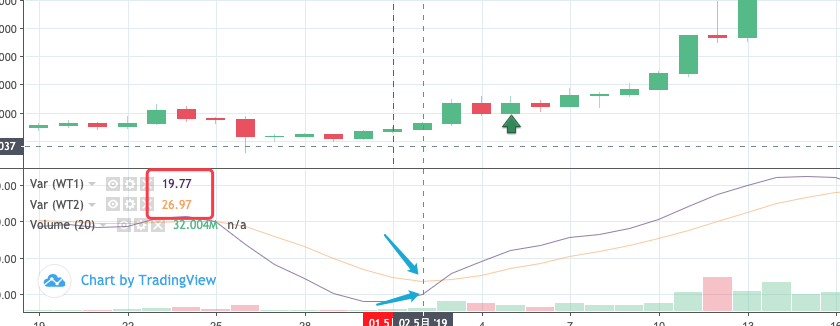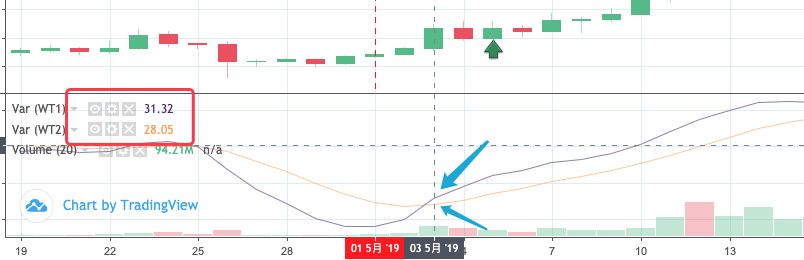``````if ((_State == IDLE || _State == SHORT) && wt1[wt1.length - 4] < wt2[wt2.length - 4] && wt1[wt1.length - 3] > wt2[wt2.length - 3]) {
if (_State == IDLE) {
_State = OPENLONG
Log("OPENLONG")    // 测试
}
if (_State == SHORT) {
_State = COVERSHORT
Log("COVERSHORT")  // 测试
}
isOK = false
}

if ((_State == IDLE || _State == LONG) && wt1[wt1.length - 4] > wt2[wt2.length - 4] && wt1[wt1.length - 3] < wt2[wt2.length - 3]) {
if (_State == IDLE) {
_State = OPENSHORT
Log("OPENSHORT")  // 测试
}
if (_State == LONG) {
_State = COVERLONG
Log("COVERLONG")  // 测试
}
isOK = false
}
``````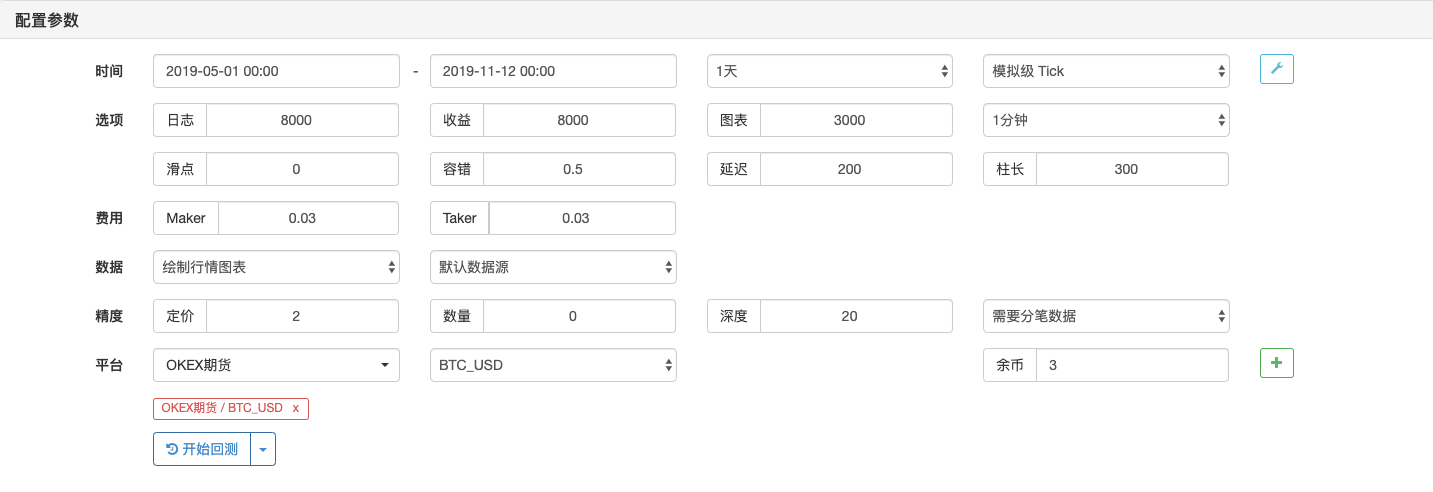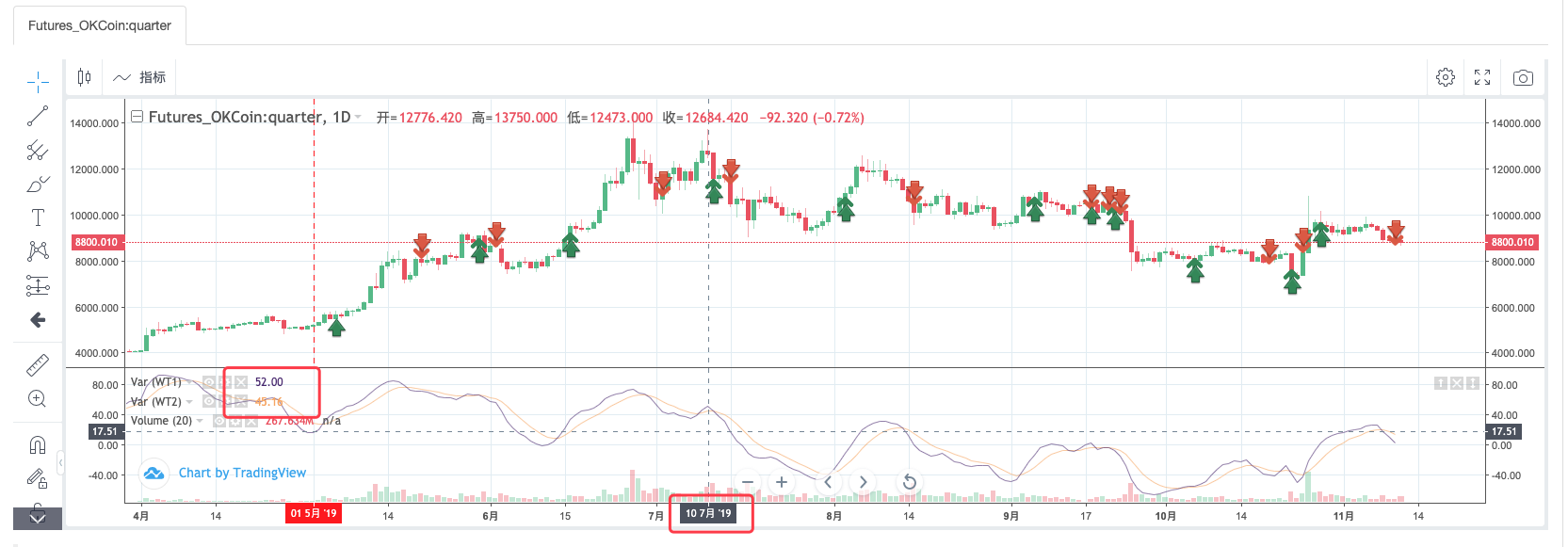JavaScript版本回测：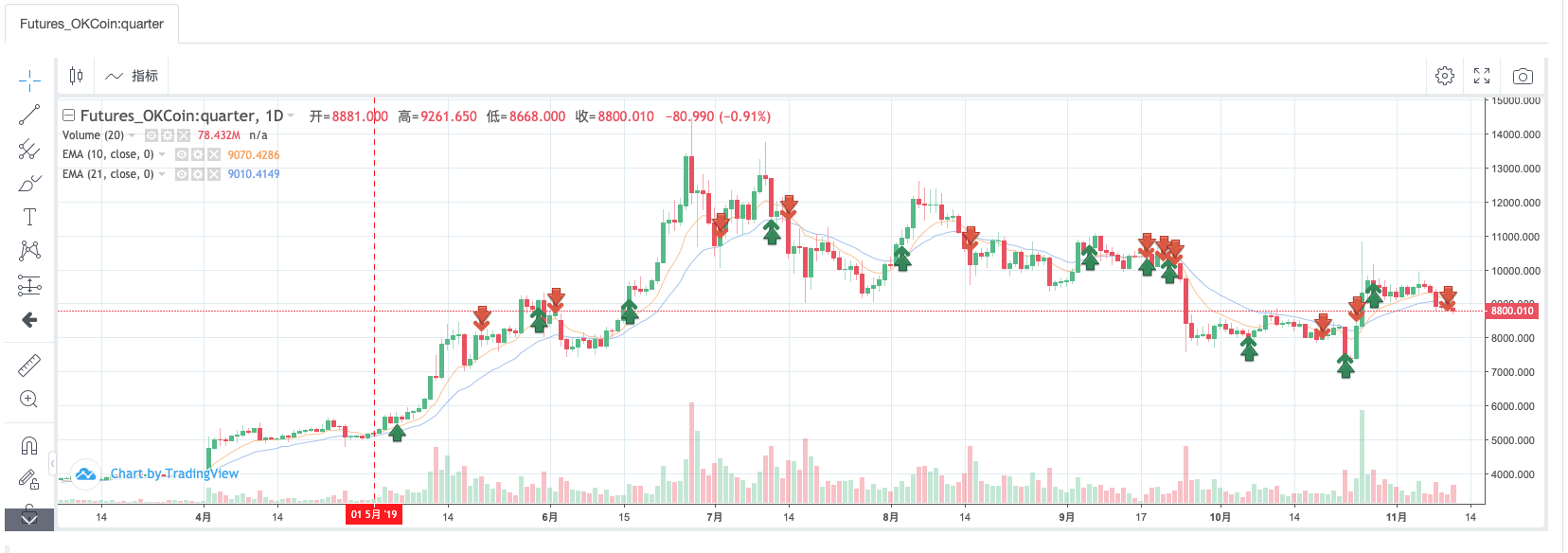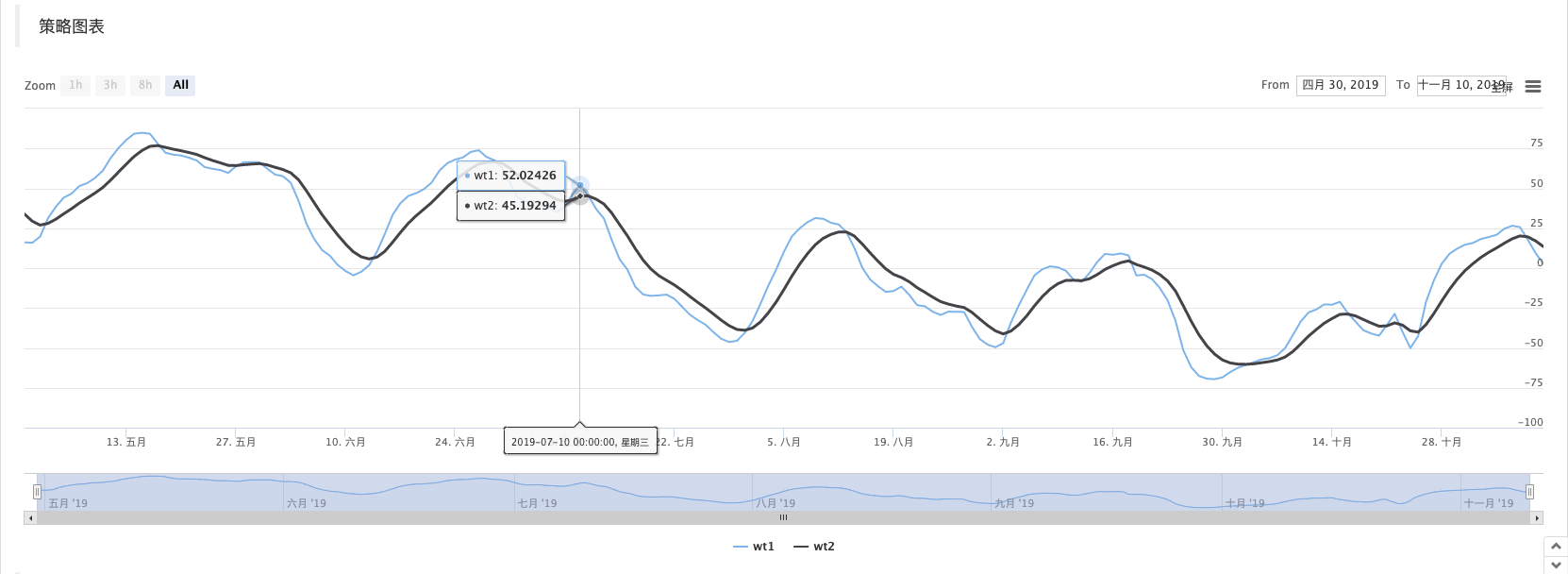OnTick函数开头部分的代码，用来让回测速度快一点，是让策略以收盘价模型来运行，有兴趣可以详细分析下。

``````function OnTick(){
// 驱动策略的行情处理部分
var records = _C(exchange.GetRecords)
if (records[records.length - 1].Time == preTime) {
if (isOK) {
Sleep(500)
return
}
} else {
preTime = records[records.length - 1].Time
}
...
..
.
``````

https://www.fmz.com/strategy/174457